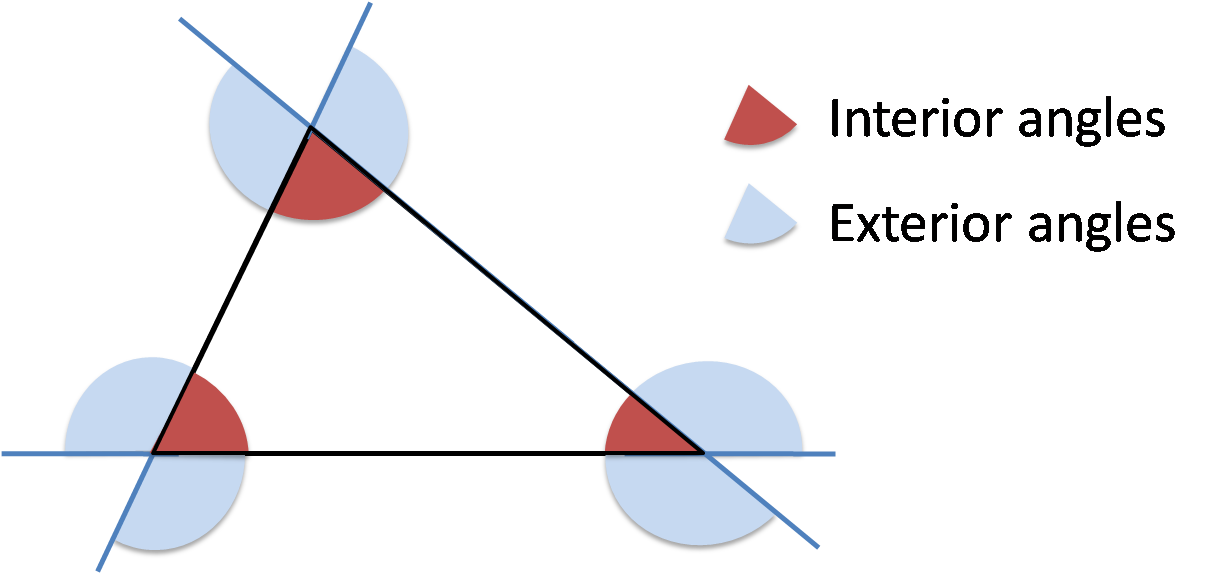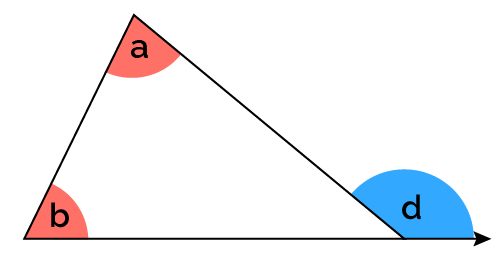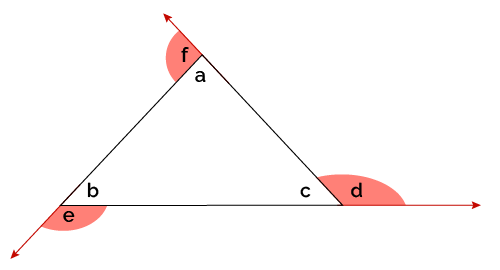# Interior and Exterior Triangle Angles Calculator

Created by Mariamy Chrdileli
Reviewed by Arturo Barrantes
Last updated: Jun 06, 2022

Welcome to the Omni interior and exterior triangle angles calculator, the convenient tool that will help you calculate the interior and exterior angles of a triangle. Are you wondering how to find the exterior and interior angles of a triangle? Then you're at the right place. Come along, and learn about the triangle sum theorem and the exterior angle theorem! 👩‍🏫

## How do I find interior angles of a triangle? Triangle sum theorem

Interior angles of a triangle are angles found inside the shape. According to the triangle angle sum theorem, sum of interior angles of a triangle always equals 180°:

a + b + c = 180°

For example, if you were trying to calculate an unknown interior angle a, you would utilize the triangle angle sum theorem in the following way:

• a = 180° - b - c (in degrees)
• a = π - b - c (in radians)

Keep on reading to learn about the definition of the exterior angle of a triangle!

## How do I find exterior angles of a triangle?What is the definition of the exterior angle of a triangle? If you were to extend any side of a triangle, the angle outside the triangle, adjacent to an interior angle, is an exterior angle of a triangle. Each triangle has six exterior angles, and two angles at each vertex are equal in value.

To find exterior angles of a triangle, you can use the exterior angle theorem. The exterior angle theorem states that the exterior angle is equal to the sum of the triangle's two opposite interior angles.For instance, if you wanted to calculate the exterior angle d, you would use the following formula:

d = a + b

## What is the sum of the exterior angles of a triangle?

The sum of exterior angles of a triangle always adds up to 360°, while the sum of interior angles of a triangle equals 180°.

## How does the interior and exterior angles calculator work?

Using the interior and exterior triangle angles calculator is very straightforward!

Just enter two of any interior angles of the triangle, and the calculator will determine all remaining unknown values of interior and exterior angles.

Don't forget to change units of measurement for angles as per your convenience!

If you find the interior and exterior triangle angles calculator helpful, check out other related tools:

• Triangle angle;
• Classifying triangles calculator;
• Triangle sum theorem calculator;
• Triangle similarity calculator;
• Exterior angles of a triangle calculator; and
• Triangle proportionality theorem calculator.

## FAQ

### How do I find the third interior angle in a triangle if two other angles are 45° and 90°?

To find the third interior angle in a triangle if two other angles are 45° and 90°:

1. Add 45 to 90; in other words, calculate the sum of two known interior angles of a triangle.
2. Take the number you computed in the previous step, and subtract it from 180.
3. Voila! The value of a third angle is 45°.

### How do I find interior and exterior angles of a triangle?

• To find an interior angle of a triangle, take the sum two known interior angles of a triangle and subtract the number from 180.
• To find an exterior angle of a triangle, simply calculate the sum two opposite interior angles of a triangle.
Mariamy Chrdileli
Interior anglesa
deg
b
deg
c
deg
Exterior angles
d
deg
e
deg
f
deg
People also viewed…

### Black Hole Collision Calculator

The Black Hole Collision Calculator lets you see the effects of a black hole collision, as well as revealing some of the mysteries of black holes, come on in and enjoy!

### Body fat

Use the body fat calculator to estimate what percentage of your body weight comprises of body fat.

### Characteristic polynomial

Characteristic polynomial calculator helps you determine the characteristic polynomial of any matrix of size 2×2, 3×3, or 4×4.

### Midpoint

Use the midpoint calculator to find out the midpoint of a line segment, which is the point that cuts the segment into two equal parts.